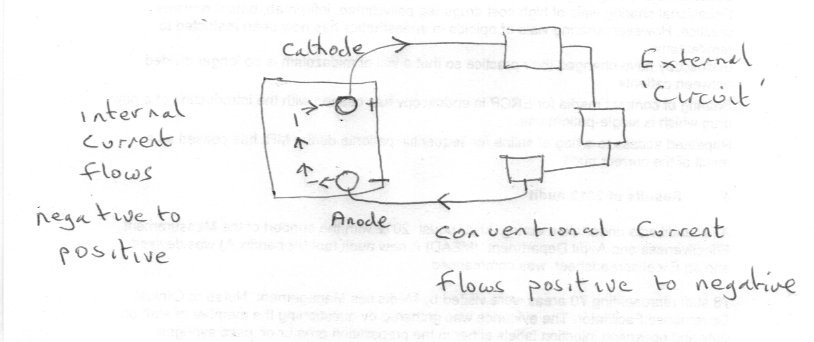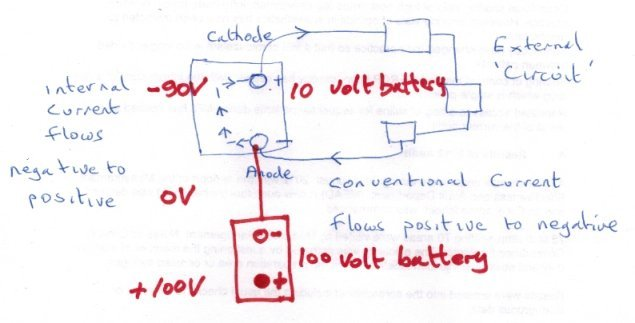# HOW BATTERIES WORK?

## Recommended Posts

Why the negative ion: OH- goes from the positive electrode to the negative electrode
in the process of using the Alkaline battery ?
t ion: OH- is negatively charged
and so is the negative electrode;
so they should repel each other.
thank you very much
ion: OH- is negatively charged
and so is the negative electrode;
so they should repel each other.
thank you very much
##### Share on other sites

14 minutes ago, ABCE said:
Why the negative ion: OH- goes from the positive electrode to the negative electrode
in the process of using the Alkaline battery ?
t ion: OH- is negatively charged
and so is the negative electrode;
so they should repel each other.
thank you very much
ion: OH- is negatively charged
and so is the negative electrode;
so they should repel each other.
thank you very much

Because the chemical reaction at the terminal marked negative on the battery is the anode and that is where electrons are released to travel around the external circuit.

In all electrical working the anode is the terminal where the electrons leave the device (in this case the battery)

The anode reaction is

Zn + 2(OH)- = ZnO + H2O + 2e-

So the electrons travel from negative around the external circuit to the positive terminal.

##### Share on other sites

1 hour ago, ABCE said:
Why the negative ion: OH- goes from the positive electrode to the negative electrode
in the process of using the Alkaline battery ?
t ion: OH- is negatively charged
and so is the negative electrode;
so they should repel each other.
thank you very much
ion: OH- is negatively charged
and so is the negative electrode;
so they should repel each other.
thank you very much

The anions move to the anode, which from the point of view of the electrolyte, has a positive charge, not a negative one, But as the anions are discharged at the anode, it means the anode becomes the source of the electrons that flow in the circuit, round to the cathode. So from the point of view of the circuit, the anode is the negative terminal of the battery.

The two points of view are opposite because in the electrolyte the electrons are moving in the direction preferred by the chemistry of the cell, while in the circuit, they flow back to where they started.

##### Share on other sites

What is exactly:"from the point of view of the electrolyte;the anode has a positive charge..."

Thanks

##### Share on other sites

Posted (edited)
21 minutes ago, ABCE said:

What is exactly:"from the point of view of the electrolyte;the anode has a positive charge..."

Thanks

That is also not right. In the electrolyte the negative charged Hydroxid ions release electrons into that electrode to be prepare to travel throw the outer circuit. So this electrode the Anode get negative charged, Oxidation takes place as described above.

Zn + 2(OH)- = ZnO + H2O + 2e-

The electrons travel thru the wire and the consumer resistor to the Plus electrode the cathode. Here a Reduction takes place  the electrons are used to reduce that material like this equation.

2 MnO2 + H2O + 2e-==> Mn2O3 + 2(OH)-

The OH- are like ferries to bring the electrons from the cathode thru the solution to the Anode.

Edited by chenbeier
##### Share on other sites

So what causes the negative ion: OH- to travel to the negative electrode?

Thanks a lot

##### Share on other sites

The Anode is made by zinc. It has an electron pressure to get dissolved. Zink eat the OH - and release the electrons into the wire., that the reason more and more OH- will sucked there. The opposit takes place at the cathode.

##### Share on other sites

The hydroxyl ions are in solution.

They are in a paste, but still in solution.

So they spread out from where they are generated and their concentration is high, (as do all ions in solution), towards where they are discharged  which happens to be the internal part of the anode.

It would be helpful if you would tell us your interest in this as we don't know how much chemistry or electric circuit theory you know.
There are some misunderstandings/mismatches between the two when you follow round a complete circuit that confuses many.

##### Share on other sites

Posted (edited)

I know more electronics and less chemistry.

Thanks a lot

So why the negaive OH- ions Wandering to the negative electrode?

please note what is the negative electrode (anode or cathode)

and the positive electrode (anode or cathode).

Thanks

Edited by ABCE
##### Share on other sites

Anode is the electrode where oxidation takes place, cathode where reduction takes place. It has nothing to do with positive or negative.

##### Share on other sites

13 minutes ago, ABCE said:

I know more electronics and less chemistry.

Thanks a lot

So why the negaive OH- ions Wandering to the negative electrode?

Thanks

First let me warn you that you have 5 posts total in your first 24 hours. So think before you use the last one and come back tomorrow if you need to.

This is not personal, just a very effective antispam measure, and you are very welcome.

OK so more electronics than chemistry.

Take a device with two terminals, like your battery, with one labelled positive and one labelled negative.
Connect up some external circuit.By itself the external circuit does not make a complete loop, although it is called a circuit.

The interesting thing is that we choose a direction of current flow (called conventional current) from positive to negative.
So the only way for a complete loop or circuit is for the current to flow from negative to positive inside the battery!

This is the reason why I defined the anode as the terminal where the electrons leave the device to the external circuit.

It is interesting to compare this with the elctronic definition of the anode and cathode for a device such as a diode.

Note the electrons flow in the opposite direction from conventional current.

OK so your question is basically how does this work out inside the battery.

This has been answered here several times actually, in different ways, but the answer is chemistry not positive-negative attraction and repulsion.

Where you have a lot of ions in solution they will spread out so that ions of the same polarity will not congregate together but try to get as far (on average) away from each other as possible. Clearly if there are lots of negative ions in solution that will also discourage further negative ions going into solution. But where the negative ions are being discharged their concentration will be below average so negative ions from the rest of the solution will migrate towards the region of discharge due to the concentration gradient. (This is what exchemist was refering to when he said that from the solution point of view the anode is actually less negative).
This will in turn reduce the concentration elsewhere, allowing more negative ions to be created at the other terminal, keeping the flow going (which is what chenbeier was referring to)

Try to follow the argument through on a diagram like mine and see if that helps.

##### Share on other sites

Posted (edited)

So why the electrons that created in the negative electrode are wadering to the positive electrode

and are not staying in the negative electrode in order to congregate together;

as the negative ions: OH- do?

Thanks a lot

Edited by ABCE
##### Share on other sites

Posted (edited)
1 hour ago, ABCE said:

So why the electrons that created in the negative electrode are wadering to the positive electrode

and are not staying in the negative electrode in order to congregate together;

as the negative ions: OH- do?

Thanks a lot

You keep referring to the positive (or negative) electrode as though positive and negative were absolute measurements.

They are not they are relative statements. Positive relative to what ? Negative relative to what ?

I have added a second battery to my sketch connecting its 'negative' terminal to the negative terminal of the first battery.
If the first battery has a voltage of 10 volts and the new battery has a voltage of 100 volts and I measure from the positive terminal of the new battery as shown then

*) The voltage at the combined 'negative' terminal is -100V and the voltage at the 'positive' terminal of the original 10V battery is - 90V

So is the positive terminal positive  or negative ?Edited by studiot
##### Share on other sites

Posted (edited)

So why the electrons that created in the negative electrode are wandering to the positive electrode

and are not staying in the negative electrode in order to congregate together;

as the negative ions: OH- do?

Thanks a lot

Edited by ABCE

## Create an account

Register a new account